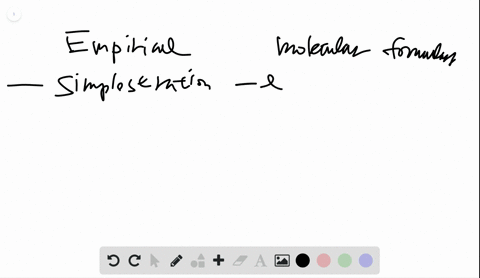Black Friday is Here! Start Your Numerade Subscription for 50% Off!Join Today### What is the difference between an empirical formu…

02:06University of Toronto
Problem 31

# Hemoglobin, a protein in red blood cells, carries $\mathrm{O}_{2}$ from the lungs to the body's cells. Iron (as ferrous ion, Fe $^{2+} )$ makes up 0.33 mass $\%$ of hemoglobin. If the molar mass of hemoglobin is $6.8 \times 10^{4} \mathrm{g} / \mathrm{mol},$ how many $\mathrm{Fe}^{2+}$ ions are in one molecule?

## Discussion

You must be signed in to discuss.

## Video Transcript

So we're keeping the hemoglobin containing iron. I am, too. So, um, for the mass percentage for Ianto off specific for iron this iron two plus 0.50% mass percentage and assumed overall more The masters exploiting eight time tender, powerful gram per mole. How many iron to ah lions getting one hemoglobin. So, first offal from Peter. We should be Ah, Richard. Okay. We should find ah, mass con tribute to the overall More the mass if you will go by and by our in. Okay, so we know that. Ah, Toto, I am, um or the mass remembers Total. I am on the mass, so we don't know how many of them. But we just know for a particular proportion off that off our mass Ah, it should be cause one to iron. So we're 6.8 times hand to allow for and they have multiply Ah, by zero point Flee Flee the white by 100 proof soup on three for yourself, a sand age So we have to divide by 100 then we should be able to find out from the course on being mass con tribute by our. So it will be 2 to 4.4 gram per bowl. It is a correspondent. Ooh, I am. But we don't know how many off them. All right, so, um, barely contain iron Embry from drop you on your table. That I am more than mass is Ah for the thief 5.85 grand for moat. So therefore ah, we can use the total mass or that's contribute by aren't divided by the mass of each kind. And then we we note the lumber off the lumber off iron, so number all fine. So you be equals throughout 2 to 4.4, do you? Why the buyer? Each off the island. And then we should have ah, um number two do for 1 44 by 55.85 and then it will be roughly around 4.0. Um, to but because we were counting harmony, number off. How many? I so it should be a whole number. So we just went down to four, 24 So therefore, in each of the whole groping